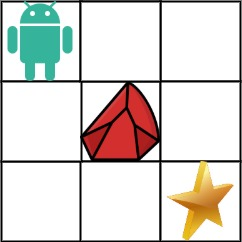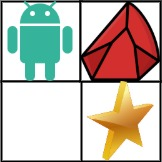# 63. Unique Paths II | LeetCode Solution

## 63. Unique Paths II | LeetCode Solution

You are given an `m x n` integer array `grid`. There is a robot initially located at the top-left corner (i.e., `grid`). The robot tries to move to the bottom-right corner (i.e., `grid[m-1][n-1]`). The robot can only move either down or right at any point in time.

An obstacle and space are marked as `1` or `0` respectively in `grid`. A path that the robot takes cannot include any square that is an obstacle.

Return the number of possible unique paths that the robot can take to reach the bottom-right corner.

The testcases are generated so that the answer will be less than or equal to `2 * 109`.

Example 1:```Input: obstacleGrid = [[0,0,0],[0,1,0],[0,0,0]]
Output: 2
Explanation: There is one obstacle in the middle of the 3x3 grid above.
There are two ways to reach the bottom-right corner:
1. Right -> Right -> Down -> Down
2. Down -> Down -> Right -> Right
```

Example 2:```Input: obstacleGrid = [[0,1],[0,0]]
Output: 1
```

Constraints:

• `m == obstacleGrid.length`
• `n == obstacleGrid[i].length`
• `1 <= m, n <= 100`
• `obstacleGrid[i][j]` is `0` or `1`.

class Solution {
public:

int helper(int a,int b,int m,int n,vector<vector<int>>&dp,vector<vector<int>>&grid){
if(a>m-1 || b>n-1){
return 0;
}
if(grid[a][b]==1)return 0;

if(a==m-1 && b==n-1){
return 1;
}

int ans=0;
if(dp[a+1][b]==-1){
dp[a+1][b]=helper(a+1,b,m,n,dp,grid);
}
if(dp[a][b+1]==-1){
dp[a][b+1]=helper(a,b+1,m,n,dp,grid);
}
ans=ans+dp[a+1][b]+ dp[a][b+1];

return ans;
}

int uniquePathsWithObstacles(vector<vector<int>>& grid) {
int n=grid.size();
int m=grid.size();
vector<vector<int>>dp(n+1,vector<int>(m+1,-1));
return helper(0,0,n,m,dp,grid);
}
};